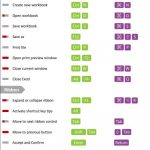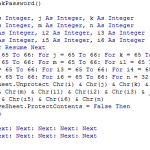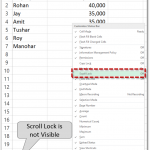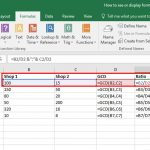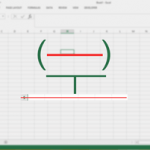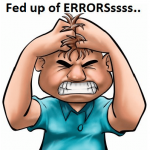Need Help? Chat with us

Vlookup Formula

### How to Use Excel VLOOKUP Formula

What is Excel VLOOKUP & How to Use? Excel VLOOKUP is an Excel function to lookup and retrieves data from a specific column in the table. VLOOKUP supports approximate and exact matching. The “V” stands for “vertical”. Lookup values must appear in the first column of the table, with lookup columns to the right. you...

### Excel VLOOKUP Function

Before we learn how to use Excel VLOOKUP function, we must learn when do we use Excel VLOOKUP function? Task: Imagine if you have to find when does train no. 6948 departs in the schedule chart above? Your approach: You will scan the first column top to bottom. When you find the same train no.,...